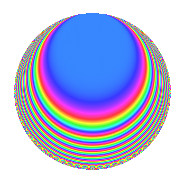Properties

 Label 3600.1.daLevel 3600 Weight 1 Character orbit da Rep. character $$\chi_{3600}(1343,\cdot)$$ Character field $$\Q(\zeta_{12})$$ Dimension 8 Newforms 1 Sturm bound 720 Trace bound 0

Related objects

Defining parameters

 Level: $$N$$ = $$3600 = 2^{4} \cdot 3^{2} \cdot 5^{2}$$ Weight: $$k$$ = $$1$$ Character orbit: $$[\chi]$$ = 3600.da (of order $$12$$ and degree $$4$$) Character conductor: $$\operatorname{cond}(\chi)$$ = $$180$$ Character field: $$\Q(\zeta_{12})$$ Newforms: $$1$$ Sturm bound: $$720$$ Trace bound: $$0$$

Dimensions

The following table gives the dimensions of various subspaces of $$M_{1}(3600, [\chi])$$.

Total New Old
Modular forms 176 8 168
Cusp forms 32 8 24
Eisenstein series 144 0 144

The following table gives the dimensions of subspaces with specified projective image type.

$$D_n$$ $$A_4$$ $$S_4$$ $$A_5$$
Dimension 8 0 0 0

Trace form

 $$8q$$ $$\mathstrut +\mathstrut O(q^{10})$$ $$8q$$ $$\mathstrut +\mathstrut 8q^{21}$$ $$\mathstrut +\mathstrut 12q^{41}$$ $$\mathstrut +\mathstrut 4q^{61}$$ $$\mathstrut +\mathstrut 4q^{81}$$ $$\mathstrut +\mathstrut O(q^{100})$$

Decomposition of $$S_{1}^{\mathrm{new}}(3600, [\chi])$$ into irreducible Hecke orbits

Label Dim. $$A$$ Field Image CM RM Traces $q$-expansion
$$a_2$$ $$a_3$$ $$a_5$$ $$a_7$$
3600.1.da.a $$8$$ $$1.797$$ $$\Q(\zeta_{24})$$ $$D_{6}$$ $$\Q(\sqrt{-5})$$ None $$0$$ $$0$$ $$0$$ $$0$$ $$q-\zeta_{24}^{7}q^{3}+\zeta_{24}^{5}q^{7}-\zeta_{24}^{2}q^{9}+\cdots$$

Decomposition of $$S_{1}^{\mathrm{old}}(3600, [\chi])$$ into lower level spaces

$$S_{1}^{\mathrm{old}}(3600, [\chi]) \cong$$ $$S_{1}^{\mathrm{new}}(900, [\chi])$$$$^{\oplus 3}$$